Updating search results...

# 32 Results

View
Selected filters:
• NC.M1.F-BF.1Conditional Remix & Share Permitted
CC BY-NC-SA
Rating
0.0 stars

CK-12's Texas Instruments Algebra I Student Edition Flexbook allows students to better utilize a graphing calculator in understanding the fundamental concepts of algebra.

Subject:
Mathematics
Material Type:
Textbook
Provider:
CK-12 Foundation
Provider Set:
CK-12 FlexBook
Author:
Jordan, Lori
12/08/2010Conditional Remix & Share Permitted
CC BY-NC-SA
Rating
0.0 stars

CK-12 Foundation's Algebra FlexBook is an introduction to algebraic concepts for the high school student. Topics include: Equations & Functions, Real Numbers, Equations of Lines, Solving Systems of Equations & Quadratic Equations.

Subject:
Mathematics
Material Type:
Textbook
Provider:
CK-12 Foundation
Provider Set:
CK-12 FlexBook
Author:
Gloag, Andrew
Gloag, Anne
11/23/2010Conditional Remix & Share Permitted
CC BY-NC-SA
Rating
0.0 stars

CK-12 Foundation's Algebra FlexBook is an introduction to algebraic concepts for the high school student. Topics include: Equations & Functions, Real Numbers, Equations of Lines, Solving Systems of Equations & Quadratic Equations.

Subject:
Mathematics
Material Type:
Textbook
Provider:
CK-12 Foundation
Provider Set:
CK-12 FlexBook
12/06/2018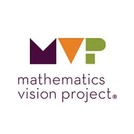Unrestricted Use
CC BY
Rating
0.0 stars

The purpose of this task is to solidify and extend the idea that geometric sequences have
a constant ratio between consecutive terms to include sequences that are decreasing (0 < r < 1).
The common ratio in one geometric sequence is a whole number and in the other sequence it is a
percent. This task contains an opportunity to compare the growth of arithmetic and geometric
sequences. This task also provides practice in writing and using formulas for arithmetic sequences.

Subject:
Math 1
Mathematics
Material Type:
Activity/Lab
Author:
The Mathematics Vision Project
02/23/2020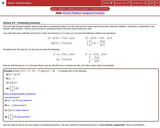Rating
0.0 stars

This website covers how to combine functions using arithmetic and also composition functions.

Subject:
Mathematics
Material Type:
Activity/Lab
Provider:
Lamar University
11/27/2017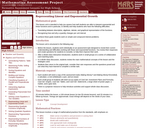Only Sharing Permitted
CC BY-NC-ND
Rating
0.0 stars

This lesson unit is intended to help teachers assess how well students are able to interpret exponential and linear functions and in particular to identify and help students who have the following difficulties: translating between descriptive, algebraic and tabular data, and graphical representation of the functions; recognizing how, and why, a quantity changes per unit intervale; and to achieve these goals students work on simple and compound interest problems.

Subject:
Algebra
Geometry
Mathematics
Material Type:
Assessment
Lesson Plan
Provider:
Shell Center for Mathematical Education
Provider Set:
Mathematics Assessment Project (MAP)
06/24/2019Unrestricted Use
CC BY
Rating
0.0 stars

This task builds upon students’ experiences with arithmetic and geometric sequences to
extend to the broader class of linear and exponential functions with continuous domains. The term
“domain” should be introduced and used throughout the whole group discussion. Students are
given contextual situations that can be modeled with either discrete and continuous linear
functions, or discrete and continuous exponential functions. They are also asked to compare these
types of functions using various representations.

Subject:
Math 1
Mathematics
Material Type:
Activity/Lab
Author:
The Mathematics Vision Project
02/23/2020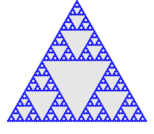Unrestricted Use
CC BY
Rating
0.0 stars

Google Sheets platform for small groups of students to record/share their discussions as they evolve their understanding of any topic, along with a Google Slides slideshow that teaches how to use the Google Sheets Group Discussion Form while small groups of students explore/discover how to generate each next row of Pascal&#39;s Triangle.

Subject:
Applied Science
Arts Education
English Language Arts
Mathematics
Science
Social Studies
Material Type:
Activity/Lab
Homework/Assignment
Student Guide
Author:
ROBERT ULMER
LORI HEATHERLY
12/14/2020Unrestricted Use
CC BY
Rating
0.0 stars

This task is to solidify understanding that geometric sequences have a constant ratio
between consecutive terms. The task is designed to generate tables, graphs, and both recursive and
explicit formulas. The focus of the task should be to identify how the constant ratio shows up in
each of the representations.

Subject:
Math 1
Mathematics
Material Type:
Activity/Lab
Author:
The Mathematics Vision Project
02/23/2020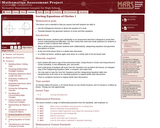Only Sharing Permitted
CC BY-NC-ND
Rating
0.0 stars

This lesson unit is intended to help teachers assess how well students are able to: use the Pythagorean theorem to derive the equation of a circle; and translate between the geometric features of circles and their equations.

Subject:
Geometry
Mathematics
Material Type:
Assessment
Lesson Plan
Provider:
Shell Center for Mathematical Education
Provider Set:
Mathematics Assessment Project (MAP)
07/31/2019Rating
0.0 stars

Lesson plan using a Cyberchase activity, students are introduced to a problem that gives them a sense of how quickly exponential growth accrues in the classic problem about a chess board and grains of rice. The context is extended to consider applications using money growth and chain letters. They are introduced to the algebraic representation for exponential growth.

Subject:
Math 1
Math 3
Mathematics
Material Type:
Activity/Lab
Interactive
Lesson Plan
Presentation
Provider:
WGBH - Teachers' Domain
Author:
WNET.org
02/26/2019Rating
0.0 stars

Class project explaining exponential decay using M&M candy

Subject:
Mathematics
Material Type:
Activity/Lab
Provider:
Weebly
01/10/2018Unrestricted Use
CC BY
Rating
0.0 stars

Sample Learning Goals
Define a function as a rule relating each input to exactly one output and predictably acting on inputs
Predict outputs of a function using given inputs
Compose functions to create a new function
Determine which functions are geometric transformations

Subject:
Mathematics
Material Type:
Activity/Lab
Author:
PhET Interactive Simulations
10/05/2021Unrestricted Use
CC BY
Rating
0.0 stars

Sample Learning Goals
Define a function as a rule relating each input to exactly one output and predictably acting on inputs
Predict outputs of a function using given inputs
Compose functions to create a new function
Interpret, compare, and translate between multiple representations of an algebraic function

Subject:
Mathematics
Material Type:
Activity/Lab
Author:
PhET Interactive Simulations
10/05/2021Unrestricted Use
CC BY
Rating
0.0 stars

The purpose of this task is to develop representations for arithmetic sequences that
students can draw upon throughout the module. The visual representation in the task should evoke
lists of numbers, tables, graphs, and equations. Various student methods for counting and
considering the growth of the dots will be represented by equivalent expressions that can be
directly connected to the visual representation.

Subject:
Math 1
Mathematics
Material Type:
Activity/Lab
Author:
The Mathematics Vision Project
02/23/2020Unrestricted Use
CC BY
Rating
0.0 stars

The purpose of this task is to develop representations for geometric sequences that
students can draw upon throughout the module. The visual representation in the task should evoke
lists of numbers, tables, graphs, and equations. Various student methods for counting and
considering the growth of the dots will be represented by equivalent expressions that can be
directly connected to the visual representation.

Subject:
Math 1
Mathematics
Material Type:
Activity/Lab
Author:
The Mathematics Vision Project
02/23/2020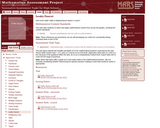Rating
0.0 stars

Students must determine how much water might a dripping faucet waste in a year. This task asks students to select and apply mathematical content from across the grades, including the content standards.

Subject:
Algebra
Math 2
Mathematics
Material Type:
Activity/Lab
Assessment
Formative Assessment
Provider:
MARS, Mathematics Assessment Resource Service
Author:
Shell Center Team
06/24/2019Only Sharing Permitted
CC BY-NC-ND
Rating
0.0 stars

This activity uses the book "One Grain of Rice" to introduce exponential functions by way of discussing hunger and famine.

Subject:
Math 1
Mathematics
Material Type:
Activity/Lab
Formative Assessment
Author:
Christy Simon
National Council of Teachers of Mathematics
11/27/2019Rating
0.0 stars

Students will draw on their foundation of the analogies between polynomial arithmetic and base-ten computation, focusing on properties of operations, particularly the distributive property. Students identify and make connections between zeros of polynomials and solutions of polynomial equations.

Subject:
Math 1
Math 2
Math 3
Mathematics
Material Type:
Unit of Study
Provider:
EngageNY
Author:
EngageNY
02/26/2019Conditional Remix & Share Permitted
CC BY-NC-SA
Rating
0.0 stars

The purpose of this task is for students to combine functions, make sense of function notation, and connect multiple representations (context, equations, and graphs). Students will also address features of functions as they solve problems that arise from this context.

Subject:
Math 1
Mathematics
Material Type:
Lesson
Author:
Mathematics Vision Project